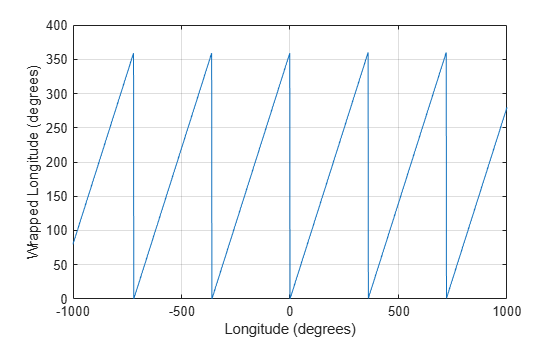# wrapTo360

Wrap angle in degrees to [0 360]

## Syntax

``lonWrapped = wrapTo360(lon)``

## Description

example

````lonWrapped = wrapTo360(lon)` wraps angles in `lon`, in degrees, to the interval [0, 360] such that 0 maps to 0 and 360 maps to 360. In general, positive multiples of 360 map to 360 and negative multiples of 360 map to zero.```

## Examples

collapse all

Specify a short list of longitudes to wrap.

`lon = [-720 -400 -360 -355 350 360 370 720];`

Wrap the longitudes to the range [0, 360] degrees.

`lonWrapped = wrapTo360(lon)`
```lonWrapped = 1×8 0 320 0 5 350 360 10 360 ```

Specify a second list of longitudes that are sampled over a large range of angles. Wrap the longitudes.

```lon2 = -1000:1000; lon2Wrapped = wrapTo360(lon2);```

Plot the wrapped longitudes. The wrapped longitudes stay in the range [0, 360] degrees.

```plot(lon2,lon2Wrapped) xlabel("Longitude (degrees)") ylabel("Wrapped Longitude (degrees)") grid on```## Input Arguments

collapse all

Angles, specified as a numeric vector.

Data Types: `single` | `double` | `int8` | `int16` | `int32` | `int64` | `uint8` | `uint16` | `uint32` | `uint64` | `logical`

## Output Arguments

collapse all

Wrapped angles, specified as a numeric vector with values in the range [0, 360].

## Extended Capabilities

### C/C++ Code GenerationGenerate C and C++ code using MATLAB® Coder™.